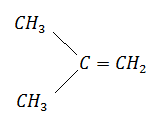Nitration of benzene is:

(a) nucleophilic substitution

(b) electrophilic substitution

Concept Questions :-

Aromatic Hydrocarbons - Benzene - Structure, Preparation & Chemical Reactions
High Yielding Test Series + Question Bank - NEET 2020

Difficulty Level:

Which of the following compounds has the lowest boiling point?

(a) ${\mathrm{CH}}_{3}{\mathrm{CH}}_{2}{\mathrm{CH}}_{2}{\mathrm{CH}}_{2}{\mathrm{CH}}_{3}$

(b) ${\mathrm{CH}}_{3}\mathrm{CH}=\mathrm{CH}-{\mathrm{CH}}_{2}{\mathrm{CH}}_{3}$

(c) ${\mathrm{CH}}_{3}\mathrm{CH}=\mathrm{CH}-\mathrm{CH}={\mathrm{CH}}_{2}$

(d) ${\mathrm{CH}}_{3}{\mathrm{CH}}_{2}{\mathrm{CH}}_{2}{\mathrm{CH}}_{3}$

Concept Questions :-

Aliphatic Hydrocarbon- physical properties
High Yielding Test Series + Question Bank - NEET 2020

Difficulty Level:

1-butyne on reaction with hot alkaline ${\mathrm{KMnO}}_{4}$ gives:

1. ${\mathrm{CH}}_{3}{\mathrm{CH}}_{2}{\mathrm{CH}}_{2}\mathrm{COOH}$

2. ${\mathrm{CH}}_{3}{\mathrm{CH}}_{2}\mathrm{COOH}$ + ${\mathrm{CO}}_{2}$

3. ${\mathrm{CH}}_{3}{\mathrm{CH}}_{2}\mathrm{COOH}$

4. ${\mathrm{CH}}_{3}{\mathrm{CH}}_{2}\mathrm{COOH}$ + HCOOH

Concept Questions :-

Alkanes, Alkenes and Alkynes - Chemical Properties
High Yielding Test Series + Question Bank - NEET 2020

Difficulty Level:

${\mathrm{CH}}_{3}-\mathrm{C}\equiv \mathrm{C}-{\mathrm{CH}}_{3}\stackrel{{\mathrm{NaNH}}_{2}}{\to }$ X; what is X?

(a) ${\mathrm{CH}}_{3}-{\mathrm{CH}}_{2}{\mathrm{CH}}_{2}{\mathrm{CH}}_{3}$

(b) ${\mathrm{CH}}_{3}{\mathrm{CH}}_{2}\mathrm{C}\equiv \mathrm{CH}$

(c)(d) ${\mathrm{CH}}_{2}=\mathrm{C}=\mathrm{CH}-{\mathrm{CH}}_{3}$

Concept Questions :-

Alkanes, Alkenes and Alkynes - Chemical Properties
High Yielding Test Series + Question Bank - NEET 2020

Difficulty Level:

Acetylene on reacting with hypochlorous acid gives:

1. ${\mathrm{CH}}_{3}\mathrm{COCl}$                                   2. ${\mathrm{ClCH}}_{2}\mathrm{CHO}$

3. ${\mathrm{Cl}}_{2}\mathrm{CH}.\mathrm{CHO}$                                4. ${\mathrm{ClCH}}_{2}\mathrm{COOH}$

Concept Questions :-

Alkanes, Alkenes and Alkynes - Chemical Properties
High Yielding Test Series + Question Bank - NEET 2020

Difficulty Level:

Benzene reacts with ${\mathrm{CH}}_{3}\mathrm{Cl}$ in the presence of anhy. ${\mathrm{AlCl}}_{3}$ to form

(a) toluene

(b) chlorobenzene

(c) benzylchloride

(d) xylene

Concept Questions :-

Aromatic Hydrocarbons - Benzene - Structure, Preparation & Chemical Reactions
High Yielding Test Series + Question Bank - NEET 2020

Difficulty Level:

$\mathrm{CH}\equiv \mathrm{CH}\stackrel{{\mathrm{O}}_{3}/\mathrm{NaOH}}{\to }$X$\stackrel{\mathrm{Zn}/{\mathrm{CH}}_{3}\mathrm{COOH}}{\to }$Y. Y is:

1. ${\mathrm{CH}}_{2}\mathrm{OH}-{\mathrm{CH}}_{2}\mathrm{OH}$                         2. ${\mathrm{CH}}_{3}{\mathrm{CH}}_{2}\mathrm{OH}$

3. ${\mathrm{CH}}_{3}\mathrm{COOH}$                                 4. ${\mathrm{CH}}_{3}\mathrm{OH}$

Concept Questions :-

Alkanes, Alkenes and Alkynes - Chemical Properties
High Yielding Test Series + Question Bank - NEET 2020

Difficulty Level:

What is the end product of the following sequences of operations?

${\mathrm{CaC}}_{2}\stackrel{{\mathrm{H}}_{2}\mathrm{O}}{\to }$A$\underset{{\mathrm{Hg}}^{2+}}{\overset{\mathrm{Dil}.{\mathrm{H}}_{2}{\mathrm{SO}}_{4}}{\to }}$$\underset{{\mathrm{H}}_{2}}{\overset{\mathrm{Ni}}{\to }}$C

1. Methyl alcohol                              2. Acetaldehyde

3. ${\mathrm{C}}_{2}{\mathrm{H}}_{5}\mathrm{OH}$                                      4. ${\mathrm{C}}_{2}{\mathrm{H}}_{4}$

Concept Questions :-

Alkanes, Alkenes and Alkynes - Chemical Properties
High Yielding Test Series + Question Bank - NEET 2020

Difficulty Level:

Alkynes can be reduced to alkenes by hydrogenation in presence of:

(a) raney Ni                              (b) anhy. ${\mathrm{AlCl}}_{3}$

(c) Pd                                       (d) Lindlar's catalyst

Concept Questions :-

Alkanes, Alkenes and Alkynes - Chemical Properties
High Yielding Test Series + Question Bank - NEET 2020

Difficulty Level:

$\mathrm{R}-\mathrm{CH}={\mathrm{CH}}_{2}\underset{{\mathrm{C}}_{2}{\mathrm{H}}_{5}\mathrm{OH}}{\overset{\mathrm{Na}/{\mathrm{NH}}_{3}\left(\mathrm{l}\right)}{\to }}$${\mathrm{RCH}}_{2}{\mathrm{CH}}_{3}$ is called:

(a) Clemmensen reduction                   (b) Fisher-Spier reduction

(c) Birch reduction                              (d) Arndt-Eistert reduction

Concept Questions :-

Alkanes, Alkenes and Alkynes - Chemical Properties